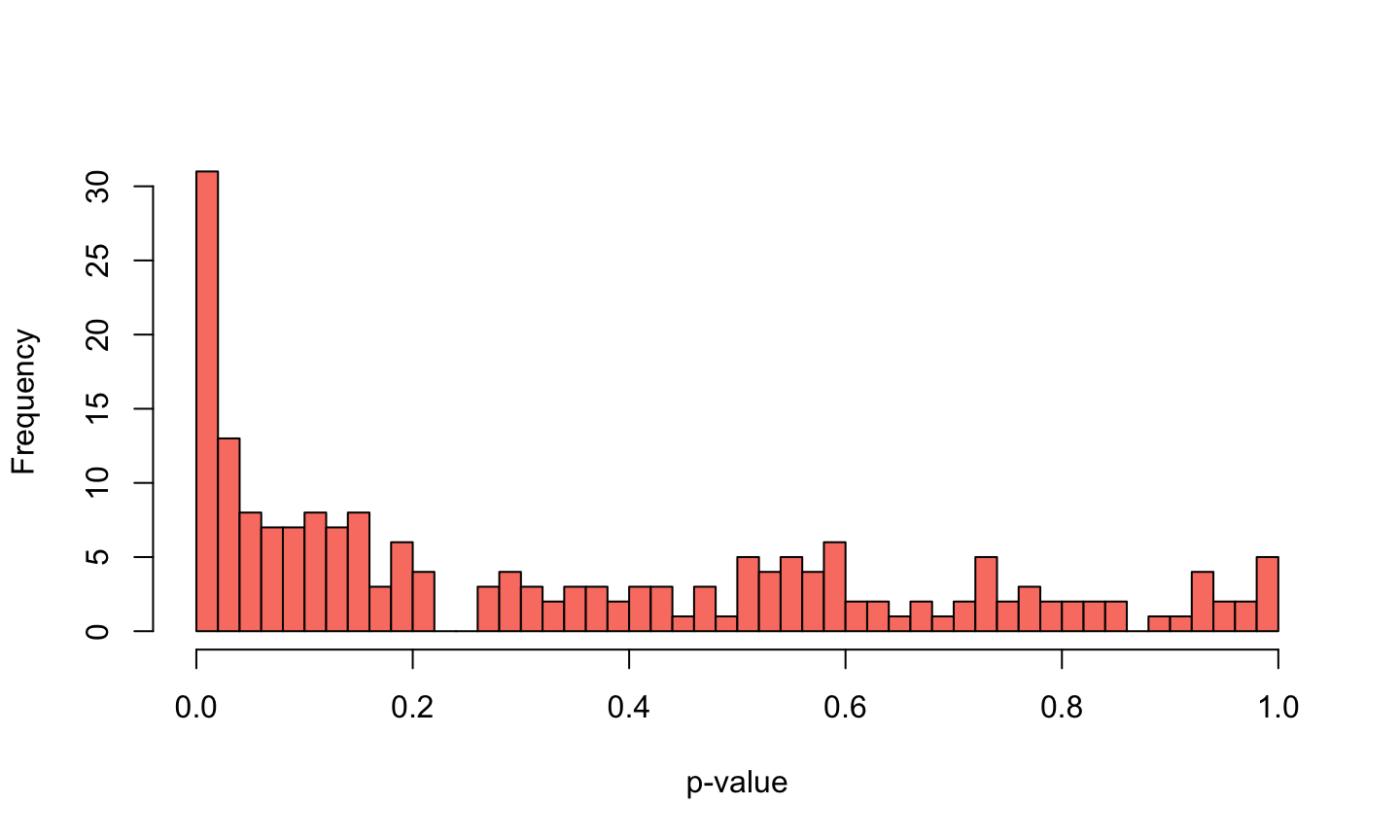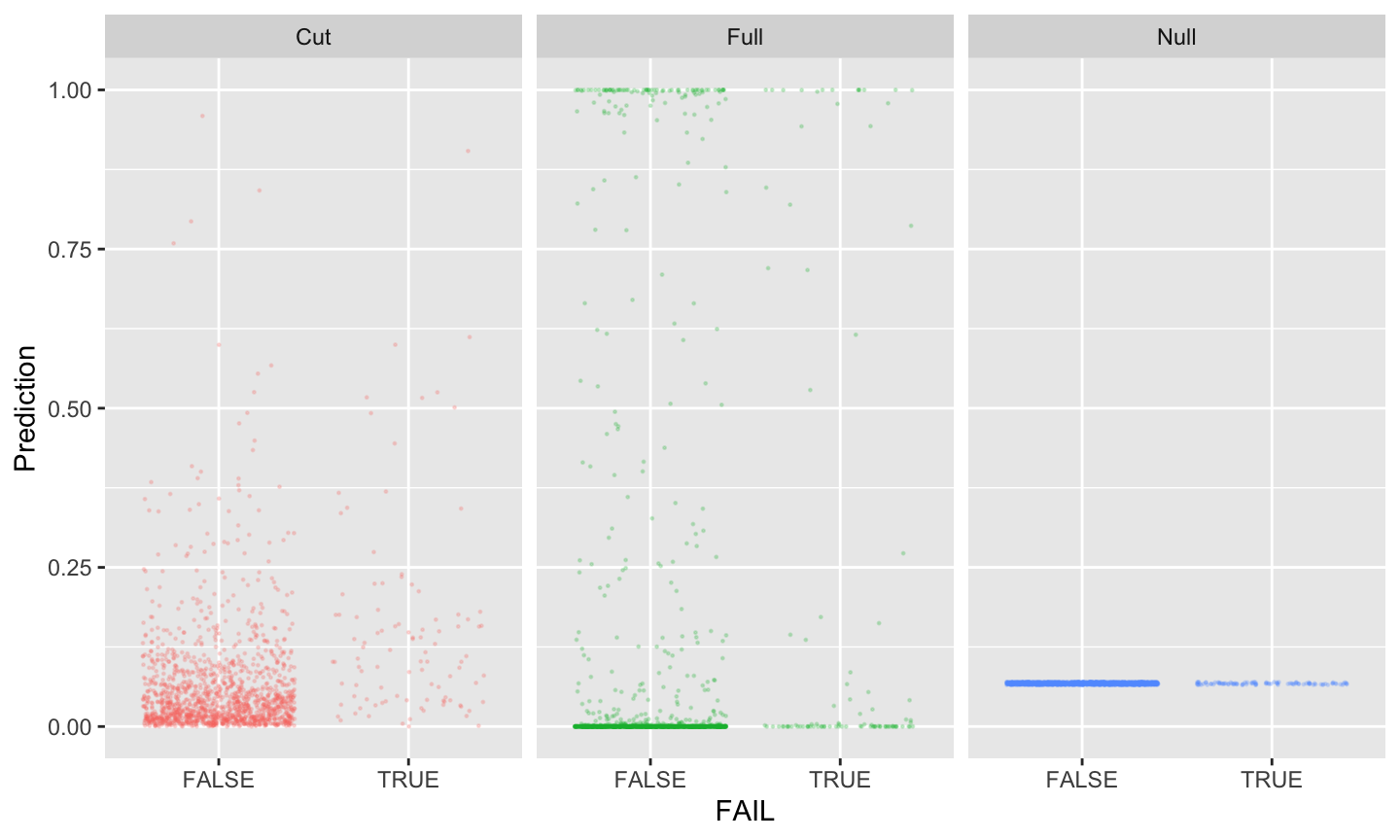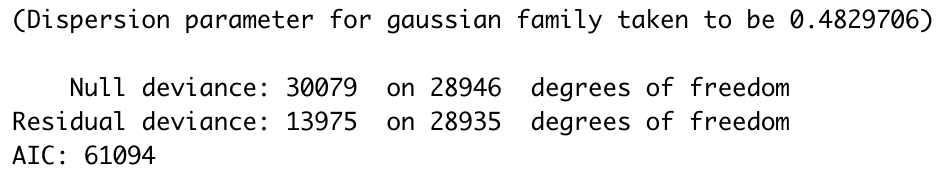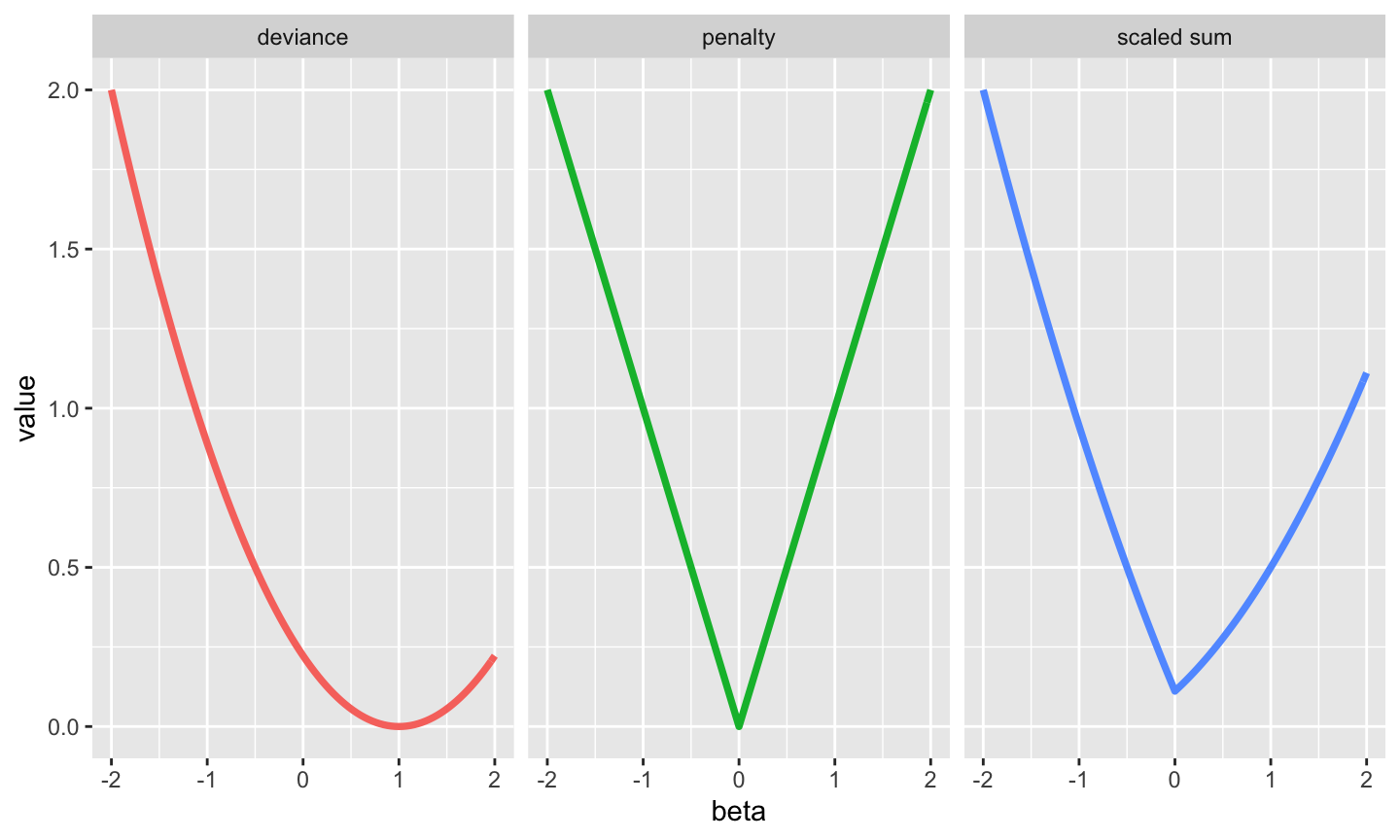April 13th, 2021

## Today’s Class

1. Quick Review
• Deviance
• Bootstrap
• Out-of-Sample
2. Comparing Models – A primer
• T-tests
• F-tests
• AIC/AICc/BIC
3. Stepwise Regression
4. Regularization and LASSO
5. Cross-Validation

## Deviance

Deviance refers to the distance between our fit and the data. You generally want to minimize it.

$Dev(\beta) = -2 log(L(\beta|data))+C$ We can ignore C for now.

Deviance is useful for comparing models. It is a measure of GOF that is similar to the residual sum of squares, for a broader class of models (logistic regression, etc). It is closely related to $$R^2$$

$R^2 = 1-\frac{D}{D_0} = 1- \frac{Dev(\hat{\beta}))}{Dev(\textbf{0})}$

## Bootstrap

Basic Procedure for data with $$n$$ observations.

1. Draw $$n$$ observations (with replacement) from your data at random, forming a new sample $$(X,Y)'$$.
2. Estimate the parameter of interest (e.g. $$\beta$$) in the sample $$(X,Y)'$$. Save that value.
3. Repeat steps (1) and (2) 5k times.
4. Use distribution of values of $$\hat{\beta}$$ to calculate p-values, CIs, etc.

## Out of Sample

We care about how well our model works out-of-sample.

One way to test this is to use a “validation sample”.

1. Randomly split your data into two samples
• usually named “testing” and “training”.
2. Fit your model using the “training” data
3. Test predictions on the left-out “testing” data.

## Out of Sample Deviances

The difference between in and out of sample deviance is about the sample.

Example Linear Regression

For In-Sample (IS) $$R^2$$, we have observations $$[\textbf{x}_1,y_1],...,[\textbf{x}_n,y_n]$$, which we use to fit $$\hat{\beta}_n$$. The deviance is: $dev_{is}(\hat{\beta}_n) = \sum_{i=1}^n (y_i - \textbf{x}_i'\hat{\beta}_n)^2$

The Out-of-Sample (OOS) $$R^2$$, we keep the same observations $$1..n$$ to estimate $$\hat{\beta}_n$$, but the deviance is calculated using $$m$$ new observations.

$dev_{oos}(\hat{\beta}_n) = \sum_{i=n+1}^{n+m}(y_i-\textbf{x}_i'\hat{\beta}_n)^2$

## Out of Sample Example 2: Semiconductors

Semiconductors manufacturing: extremely complicated, no margin for error, hundreds of diagnostics.

We want to focus on diagnostics that are predictive of failure.

$$\textbf{x}_i$$ is a vector of 200 diagnostic signals, $$y_i$$ is a binary (Pass/Fail) for a chip batch. There are 100 failures out of 1477 batches.

Logistic regression for failure of chip $$i$$ is $p_i = P[fail_i|\textbf{x}_i] = exp(\alpha+\textbf{x}_i' \beta)/(1+exp(\alpha+\textbf{x}_i'\beta))$

## Example 2: Semiconductors

Full model (non-interacted, jointly estimated) has an in-sample $$R^2 \approx 0.56$$ (based on binomial deviance).## Semiconductor + FDR

We could consider using FDR to slim down our model. $$q=0.1$$ yields $$\alpha \approx 0.012$$ cutoff. That leaves 25 variables, of which ~2.5 are “false”.

A ‘cut’ model using only those 25 variables has an $$R^2 \approx 0.18$$.

summary(cut.pvals)
##     Min.  1st Qu.   Median     Mean  3rd Qu.     Max.
## 0.000000 0.001762 0.030080 0.086693 0.092763 0.495039

But now we have a bunch of large pvalues again. One question: Should we repeat this process?

## Semiconductors Cont.

We care about out of sample performance. Let’s examine it.

In Sample $$R^2$$

• $$R^2$$ increases with more covariates.
Larger model $$\implies$$ more flexible model $$\implies$$ better in-sample fit.
• Thus, we shouldn’t use in-sample $$R^2$$ for model comparisons.

Out of Sample $$R^2$$.

• “How well can this model predict data it hasn’t seen?”
• K-Fold Cross-validation will help us estimate the out-of-sample predictive performance. Details in 30(ish) slides.

## OOS performance

We may gain predictive accuracy by dropping variables.

The OOS $$R^2$$ for the cut model: 0.10. (~50% of in-sample $$R^2$$)

The OOS $$R^2$$ for the full model: -5.87.

THIS IS NEGATIVE. Out-of-Sample, we would be much BETTER off predicting the mean than with the full model.

Estimated by using our testing-training split method – AKA cross-validation.

## Fit plotsIN SAMPLE $$R^2$$ MISLEADS US

## OOS

• In-sample measures of model fit can be misleading.
• More variables not necessarily better for out of sample performance

Cross validation uses OOS experiments to choose the best model. This is a big part of big data

Selection of ‘the best’ model is at the core of big data. We are moving into new territory here.

Before getting to selection, we need to come up with a good set of candidate models to choose from.

HOW?

## How many models are we considering?

Suppose for the time being, we ignore interactions between variables, and nonlinearities. We are just interested in models which include some subset of our variables. With $$p$$ variables, how many models are there?

• $$2^p$$
• For small numbers of variables, like 20, this is “only” ~1million model choices
• But it grows rapidly. With 200 variables, there are $$\approx 2\times 10^{60}$$ models.
• If a computer can estimate 1 trillion/second, and we have 1 trillion computers, it would take more years than there are atoms in a human body to estimate every regression.
• So we will need to ignore a few of those

## T-tests/FDR

T-tests are the source for the p-values we’ve been using for FDR control.

We could (as we did above), estimate the full joint model (or many marginal models) and use the pvalues from these t-tests to choose our covariates, ditching the covariates that are insignificant, either under standard rejection or FDR control frameworks.

But as we saw, the p-values shift as we change models. Do we iterate?

There are other problems, like independence of p-values, data with $$p>n$$ where we can’t estimate p-values, and covariates that are useful only with or without other variables.

Is “variable significance” really what we care about?

## Stepwise Regression

Stepwise regression is an important historical model building technique. Once we accept that we can’t estimate every subsetted model, we ask “what if we start with an empty model and slowly build it up?”

Stepwise Algorithm (Forward)

2. Enumerate all models which contain 1 more variable.
3. Estimate those models
4. Compare each of those models to the current model.
5. Pick the best model, and make it the current model.
6. Repeat steps 2-5.

When to stop? Stop when your model selection rule prefers the current model to all candidate models. Model selection rules in a moment.

## Stepwise Regression

This is a useful heuristic search procedure, which reduces the problem space significantly. $$\implies$$ can be easy to estimate.

There are many variants

• Backwards: start with some maximal model, and shrink it at each step
• Bidirectional: Start with some model, compare to all models one parameter smaller or larger at each step
• Large step size: Check all models with 2 variables more (or 10, etc) at each step

How do we choose?

## F-tests

There is a standard tool for comparing two (nested) models in linear regression. The “F-test”.

The F-test is purely a function of the $$R^2$$ and the number of variables.

You could build a procedure out of iteratively comparing two models.

But this will again mostly be selecting mostly for variables which are “significant” rather than optimizing for our predictive loss.

Again, this is not really what we want.

## AIC

What we care about is Out-of-Sample predictive performance.

It turns out (after a lot of theoretical work) that we can estimate that using Akaike’s Information Criterion. For a model $$M$$ with $$k$$ variables estimated to be $$\hat{\beta}_M$$

$AIC = 2k + Dev(\hat{\beta}_M) = 2k - 2 log(L(\hat{\beta}_M|data))$ This was in our basic model output.## AIC

The AIC actually estimates OOS deviance – what your deviance would be in another independent sample of size $$n$$.

In-sample deviance is too small, because the model minimizes that. Some incredible theory work shows us that $$AIC \approx OOS\ Deviance$$.

This approximation is good when $$\frac{n}{k}$$ is very big.

In big data, that may not apply. Often $$k \approx n$$ or is bigger. In that case, AIC overfits!

We want to minimize the AIC.

• AICc
• BIC

## AIC corrected: AICc

AIC struggles when the number of parameters is too big.

An improved approximation is:

$AICc = Dev(\hat{\beta}_M) + 2k \frac{n}{n-k-1}$

Notice that when $$\frac{n}{k}$$ is big, $$AICc \approx AIC$$. So we will generally prefer AICc. Nptice also that it is easy to calculate AICc given AIC.

## Bayesian Information Criterion: BIC

$BIC = Dev(\hat{\beta}_M) + k\ log(n)$

This looks like AIC (swapping a $$log(n)$$ for a $$2$$) but it comes from a very different place.

$$BIC \approx -log(P[M|data])$$, the probability ‘model b is true’, in a bayesian setting.

Generally BIC will select for smaller models. Why?

Imperfect Rule of Thumb:
“AIC/AICc approximate deviance, BIC approximates truth”

## Stepwise

Stepwise regression is not choosing the globally best subset of predictors.

Remember there are $$2^k$$ such models

Instead, it takes a “greedy” approach. Looking at the best option for the immediate step, and taking that. This is computationally much easier – but isn’t likely to give you the ‘globally’ optimal model.

Its computationally easy?

## Stepwise in R

null = glm(FAIL~1,data=SC,family=binomial)
full = glm(FAIL~.,data=SC,family=binomial)
stepwise = step(null,scope=formula(full),direction="forward",trace=0) #Default is AIC
length(coef(stepwise))
##  63

Full is the largest model you would consider, and scope is it’s formula. Feel free to include interactions to make it huge.

But be warned, even though stepwise is faster than ennumerating every possible model, it is not necessarily ‘fast’.

## Variable Selection Thus Far

Subset Selection Enumerate every possible model $$M$$, estimate $$\hat{\beta}$$ for each model, choose the best.
$$\implies$$ Completely infeasible even for small $$p$$ like 50.

Stepwise Selection: A faster heuristic. Start with some base model, find the best ‘adjacent’ model, change to that, and continue iterating.

• Still not very fast (90+ seconds for semiconductor example)
• Not very stable. Small changes in a few observations can change your selected model a lot. With resulting big effects on your predictions.
• Inferential properties are extremely unclear.

What’s next? Regularization

## Regularization

The general idea: Impose restrictions on $$\hat{\beta}$$ to make them stable, purposeful, and quick to estimate.

Variable selection is achieved with Estimation, not p-values.

What are our goals?

• We would like $$\hat{\beta}$$ to be stable relative to small changes in data
• We would like $$\hat{\beta}$$ to give the best out of sample predictions possible
• We would like $$\hat{\beta}$$ to set some coefficients to exactly 0 (dropping variables from the model)

We will penalize solutions $$\hat{\beta}$$ that do not meet expectations.

## Regularization

Using all predictors $$x_1,...,x_p$$, the most likely (MLE) values $$\hat{\beta}_{MLE}$$ minimize deviance.

$\hat{\beta}_{MLE} = \underset{\beta}{\text{argmin}}\ Dev(\beta) = \underset{\beta}{\text{argmin}} -2log(L(\beta))/n$

But $$\hat{\beta}_{MLE}$$ can only be estimated when $$p < n$$, may be unstable, and won’t have exact 0s.

The penalized version of this adds a penalty $$pen()$$ which depends on the parameter $$\beta$$ and is scaled by some weight $$\lambda > 0$$.

$\hat{\beta}_{PMLE} = \underset{\beta}{\text{argmin}} \left(Dev(\beta) + \lambda\ pen(\beta) \right)$

## Regularization

This penalty imposes a cost for solutions that don’t meet our preferences. $$\implies \hat{\beta}_{PMLE}$$ is biased.

What costs should we impose? What costs do we face?

• Estimation costs: This is the deviance. As our model fit degrades, we penalize the model.
• Testing/Measurement costs: Having many parameters in the model is costly. We should penalize coefficients that aren’t $$0$$.
• A natural penalty then: $$pen(\beta) = \sum_{j=1}^p 1(\beta \neq 0)$$.
• This is just best subset selection again though. We can’t do it.
• OOS costs: Predictions near the unconditional mean of the data are ‘safe’. Big coefficients push us far away from those predictions. So we should penalize big coefficients.

## LASSO

LASSO is the most commonly used regularized (or penalized) regression model. The lasso penalty is the $$l_1$$ norm of our parameters: $$pen(\beta) = \sum |\beta_j|$$, which penalizes larger coefficients and non-zero coefficients. So our estimates are: $\hat{\beta}_\lambda = \underset{\beta}{\text{argmin}}\left( Dev(\beta)+\lambda \sum_{j=1}^p |\beta_j|\right)$

For linear regression this simplifies dramatically:

$\hat{\beta}_\lambda = \underset{\beta}{\text{argmin}}\left(\sum_{i=1}^n (y-x'\beta)^2+\lambda \hat\sigma^2\sum_{j=1}^p |\beta_j|\right)$

## LASSO

LASSO gives sparse solutions. It imposes a ‘spiky’ penalty for coefficients. The ‘spike’ at 0 yields a preference for coefficients that are 0. Non-zero coefficients need to be sufficiently informative to pay the penalty.

Even though the deviance (the other part of our maximation) is smooth, a smooth function plus a spiky one can be spiky. And the minimum can be at that spike.

## LASSOThe LASSO ‘shrinks’ some coefficients to 0.
$$\implies$$ Automatic variable selection.

This is why LASSO is sometimes referred to as modern least squares’.

## LASSO Problems

But LASSO also shrinks the parameters that wind up being non-zero.

In particular, it can be shown that for a given $$\lambda$$,

$\hat{\beta}_{j,\lambda} = \hat{\beta}_{j,OLS}\ \text{max}\left(0,1-\frac{n\lambda}{|\hat{\beta}_{j,OLS}|}\right)$ So when $$\hat\beta_{j,\lambda}$$ is non-zero, it is $$\approx n\lambda$$ closer to 0 than $$\hat\beta_{j,OLS}$$.

This, as well as the zeroing out of coefficients, induces the bias.

## LASSO in R

Very easy to estimate in R, thanks to numerous useful packages.

library(glmnet)
mod = glmnet(as.matrix(SC[,-1]),SC\$FAIL, family=binomial) #~1 seconds
sum(coef(mod, s=0.01) != 0) #s = 0.01 is setting lambda
##  53

So 147 coefficients are 0 with $$\lambda=0.01$$.

## Other Regularizations

We chose the penalty to be the sum of absolute values of $$\beta$$. But there are other choices.

• Ridge regression: the $$l_2$$ norm: $$pen(\beta) = \sum \beta^2$$
• This doesn’t have a spike at 0, so it doesn’t auto-select.
• Elastic Net: $$pen(\beta) = \gamma \sum |\beta| + (1-\gamma)\sum \beta^2$$
• This is ‘between’ LASSO and Ridge. It does some variable selection.
• Many more: ‘spike and slab LASSO’, ‘gamma LASSO’, etc

## LASSO Details

LASSO finds $$\hat{\beta}_\lambda$$ which minimizes $$Dev(\beta)+\lambda\sum|\beta|$$. How do we pick $$\lambda$$?

R will estimate a sequence of $$\lambda_1 < \lambda_2 < ... < \lambda_T$$. We can apply model selection tools to choose the best $$\hat\lambda$$

LASSO path estimation: R does the following:

1. Start with small $$\lambda_1 \approx 0$$ such that $$\hat\beta_{\lambda_1} \approx \hat\beta_{MLE}$$
2. Choose $$\lambda_2$$, compute $$\hat{\beta}_{\lambda_2}$$.
3. Keep increasing $$\lambda$$ until you obtain the null model for some value, $$\lambda_T$$
• This is fast. Each update is easy.
• This is stable, optimal lambda may change slightly between samples, but not much.
• This is an improved ‘stepwise’ regression.

## Classic Plot

Each lambda has a different series of coefficients. There is a classic plot illustrating. plot(mod)`